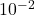wo parallel conducting plates are separated by 10.0 cm, and one of them is taken to be at zero volts. (a) What is the magnitude of the elect

Question

wo parallel conducting plates are separated by 10.0 cm, and one of them is taken to be at zero volts. (a) What is the magnitude of the electric field strength between them, if the potential 7.05 cm from the zero volt plate (and 2.95 cm from the other) is 393 V?

in progress 0
5 months 2021-08-27T13:08:11+00:00 1 Answers 2 views 0

-18896.49 V/m

Explanation:

Distance between the two plates = 10 cm = 10 xm = 0.1 m

Also, one of the plates is taken as zero volt.

a. The potential strength between the zero volt plate, and 7.05 cm (0.0705 m) away is 393 V

b. The potential strength between the other plate, and 2.95 cm (0.0295 m) away is 393 V

Potential field strength = -dV/dx

where dV is voltage difference between these points,

dx is the difference in distance between these points

For the first case above,

potential field strength = -393/0.0705 = -5574.46 V/m

For the second case ,

potential field strength = -393/0.0295 = -13322.03 V/m

Magnitude of the field strength across the plates will be

-5574.46 + (-13322.03) = -5574.46 + 13322.03 = -18896.49 V/m